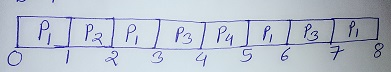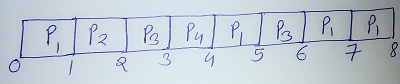# Net 39

CBSE NET DECEMBER 20133 PAPER II

OPERATING SYSTEM

Q. Consider a pre-emptive priority based scheduling algorithm based on dynamically changing priority. Larger priority number implies higher priority. When the process is waiting for CPU in the ready queue (but not yet started execution), its priority changes at a rate a = 2. When it starts running, its priority changes at a rate b = 1. All the processes are assigned priority value 0 when they enter ready queue. Assume that the following processes want to execute:
 Process ID Arrival Time Service Time P1 0 4 P2 1 1 P3 2 2 P4 3 1
The time quantum q = 1. When two processes want to join ready queue simultaneously, the process which has not executed recently is given priority. The finish time of processes P1, P2, P3 and P4 will respectively be

(A) 4, 5, 7 and 8
(B) 8, 2, 7 and 5
(C) 2, 5, 7 and 8
(D) 8, 2, 5 and 7

Ans: (B)

Explanation: When priority changes at a rate a=2, b=1, Gantt Chart :So from above Gantt Chart,
Finish time of P1=8,P2=2,P3=7,P4=5.

If we do not consider priority changes than Gantt Chart become:So from above Gantt Chart,
Finish time of P1=8,P2=2,P3=6,P4=4.